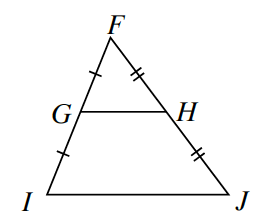### Home > INT1 > Chapter Ch10 > Lesson 10.2.2 > Problem10-95

10-95.

Examine the diagram at right. $ΔFGH$and $ΔFIJ$are similar. If $GH = 4x – 3$ and $IJ = 3x + 14$, write an equation and solve for x. Then calculate the length of $GH$.Since $G$ is the midpoint of segment $FI$ and $H$ is the midpoint of segment $FJ$, segment $IJ$ is twice as long as $GH$.